Next: Bimolecular rates Up: OPTIM User Guide Previous: Auxiliary Programs   Contents

# Calculating (Quantum) Rates and Instanton Search

The following is a user documentation on (a) classical rate calculations, (b) getting the initial files for instanton optimisations, (c) optimise instantons, (d) calculate instanton rates. The procedure follows the one as implemented in the programm package DL-find. More details about the algorithms can be found here [83,36]. Note that for the interface to work properly one has to set the number of frozen atoms in odata and provide there a geomtry of one image as well to obtain the proper masses of the system. Before starting generate a executable with ./build.csh dlfoptim ifort. The ifort compiler is recommended to be used.

1. Location of a minima and a saddle points on the potential energy surface After locating the classical minima and transition states choose a pair of them such that there is no barrier between the saddle point and the minimum. The next step is to obtain the initial input files for the instanton rate calcualtions based on Im F theory. Copy one of the two chosen geometries to the file 'input.xyz' (in xyz format and with atom names). This has to be done for both the reactant and the transition state. By setting the keyword INSTANTONSTARTDUMP the files qts_reactant.txt and qts_hessian_rs.txt in case of the reactant, and qts_ts.txt and qts_hessian_ts.txt in case of the transition state are written. In case of a TS, the crossover temperature Tc is calculated:

 Tc =(8)

with ωb being the absolute value of the imaginary frequency. Here is an example odata file:

 NIMET FREEZERANGE 296 393 INSTANTONSTARTDUMP 300 POINTS etc.

2. Classical rates (with tunnelling corrections): the files qts_reactant.txt, qts_ts.txt, and class.in have to be provided. The latter is an input file of the following format:
first line: ignored
second line: number of zero eigenvalues for the reactant and for the TS (e.g. 6 6'')
third line: starting temperature, end temperature, number of temperature steps (e.g. 300. 150. 20).
fourth line: T'' if bimolecular rates should be calculated, see below (14.1 on page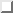). Here is an example file:

 393 393 0 0 200. 50. 10 F

These files are required to run a classical rate calcualtion with CLASSICALRATES in OPTIM through an interface to the quantum rates module in DL-FIND. The output (stdout or the file arrhenius) can directly be used in an Arrhenius plot: 1000/T in Kelvin, log10 of the classical rates in s-1 (cm3s-1 in case of bimolecular rates) calculated completely classical, with quantised vibrations (which includes the zero-point vibrational energy) and including tunnelling approximatively via the simplified Wigner correction:

 κ(T) = 1 +(β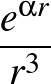ωb)2 = 1 +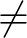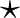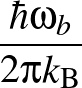,    κ(Tc) = 1 +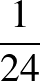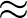2.645 (9)

For temperatures above the crossover temperature Tc, the full Wigner-corrected rates is also given:

 κ(T) =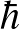(10)

In the last column the exact analytical quantum rates for a symmetric Eckart barrier fitted to the particular system (barrier hight and ωb) are shown. All degrees of freedom perpendicular to the reaction coordinate are approximated as quantum mechanical harmonic oscillators.

KIEs can be calculated directly by first running OPTIM with the keyword CLASSICALRATES instead of INSTANTONSTARTDUMP on the Hessians for the light isotopologue. The file arrhenius of this run can be copied to the file rate_H in the directory where the rate with heavier isotopes is to be calculated. There, the same class.in as in the light case (at least the same temperature parameters) should be used. The rates obtained with the light nucleids are read and the KIE is directly calculated and written to a file called kie.

3. Optimisation of the first instanton starting from the classical TS: The file qts_hessian_ts.txt has to be renamed to qts_hessian.txt. All geometrical data are read in from qts_hessian.txt. However, coordinates in input.xyz and the odata file still have to be provided (for historic reasons, number of atoms, ...), but are ignored (as in all instanton optimisations and rate calculations).

The keyword INSTANTONOPT nimageinst distortinst deltainst temperature in OPTIM specifies the optimisation of instantons.

A finite value of distortinst specifies how far the images will be spread along the unstable mode of the classical TS, see. Newton–Raphson optimisation is recommended[83,36] and currently called from OPTIM. The NR optimiser is modified to avoid convergence to higher-order saddle points . This avoids the collapse of the instanton path to the classical TS.

Instanton searches are performed in mass-weighted coordinates with masses consistent with atomic units (electron mass, me). That is, the mass of a hydrogen atom (1H) is 1837.15 me. This scales all distances up by a factor of 42.695 ( = (atomic mass unit/me)1/2) compared to mass-scaled coordinates. Thus, the tolerance criterion (tolerance) has to be smaller by the same factor to achieve equivalent convergence. A tolerance of 10-7 is usually sufficient, a tolerance of 10-8 is also often still possible. Since NR converges quadratically, the more stringent tolerance generally does not increase the number of steps dramatically.

If NR (or P-RFO) is used, the updated Hessian will be used to calculate a preliminary estimate of the rate (if qts_reactant.txt is available). In that case, qts_hessian_upd.txt will be written, which contains only the updated Hessian. qts_coords.txt will in any case be written. It acts as input for subsequent recalculation of the Hessian and a rate calculation.

Restarting instanton searches: Proper restart information (check files) is not written for the time being. Using NR, a restart is possible, though, by renaming qts_hessian_intermediate.txt (which is written after each step) to qts_hessian.txt and starting the simulation again. It will start from the Hessian and the geometry after the last full set of energies has been obtained.

4. Instanton rate calculation: First one needs to copy the file qts_hessian_intermediate.txt to qts_hessian.txt. qts_coords.txt from a previous instanton optimisation is read (input.xyz and the coordinates in odata are ignored). The temperature is also read from qts_coords.txt. The rate calculation is chosen by putting INSTANTONRATE nimageinst in the odata file. Hessians (no updates) at all images and the rate are calculated as described in . qts_hessian.txt is written, which acts as input for subsequent instanton optimisations.

Restarting of rate calculations is also only possible by using the Hessian information written for each image in qts_hessian_imageX.txt. For these files to be read, set inithessian=6. However, this requires at the moment a manual adjustment in the interface file to OPTIM.

5. Next instanton optimisation in sequential cooling: Starting from qts_hessian.txt at a previous (in general higher) temperature, another instanton is calculated. Distort should be zero, all other parameters are the same as in 3. The number of images may be increased. For optimal interpolation, the number of new images Pn should be related to the number of old images Po by:

 Pn = k Po - k + 1 (11)

with k > 1 being an integer. This ensures k - 1 new images between each pair of old images.

6. Instanton KIEs can be calculated by starting out from a Hessian (qts_hessian.txt) for a different isotopologue and changing the masses in the input. The Hessian will be re-weighted accordingly. The instanton geometry has to be re-optimised. The file qts_reactant.txt obtained with changed masses can not be used. Instead, a file qts_hessian_rs.txt (which includes the masses, so from a recent version of DL-FIND) can be provided. The Hessian of the reactant obtained from that file will also be re-weighted.

In an approximation (FPA) one can keep the instanton geometry fixed and just change the masses . This is done by calculating an instanton rate with inithessian=5 (read the Hessian from file rather than recalculating it) and changing the masses.

For lower temperature (compared to Tc) the number of images necessary can be kept at bay by adapting the integration grid (dtau) to the potential energy along the instanton path . This only makes sense if the instanton path has reached the reactant minimum. It can be achieved by setting nebk=1 in interfacetoOPTIM.f90 (this is not interpreted as the NEB force constant, but as a parameter which can vary from 0 to 1. One corresponds to a fully adaptive grid).

If KIEs should be calculated with INSTANTONRATE it is not necessary to recalculate the Hessian for the heavier isotopologue. The Hessians for the reactant and product are read in. If the masses provided via the calling code (OPTIM) are different from the ones in the Hessian files, the Hessians with the new masses will be calculated.

SubsectionsNext: Bimolecular rates Up: OPTIM User Guide Previous: Auxiliary Programs   Contents